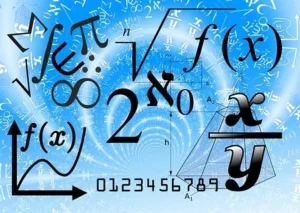September 29, 2023

# Math Formulas## Mathematical formulas :

The best approach to make mathematics interesting is to use formulae. Learning arithmetic formulae will therefore help kids build confidence and improve their problem-solving abilities. Students can improve their scores on board exams and entrance exams by using math formulae, which also make  preparation quick and simple.  For students getting ready for board exams and competitive exams, math formulas are  very essential. Algebra, calculus, geometry, and other math formulas are included in this article.

Math formulae are effective tools for expressing mathematical concepts, relationships, and calculations in a short and exact manner. These formulas provide the foundation of several mathematical fields, including algebra, geometry, calculus, statistics, and others. They provide a standardised vocabulary through which mathematicians, scientists, engineers, and researchers can efficiently communicate and solve complicated problems.

Formulas are essential in algebra for expressing relationships between variables. They give a foundation for solving equations, modifying expressions, and simplifying difficult mathematical statements. The quadratic formula, for example, is commonly used to identify the roots of a quadratic equation, whereas the Pythagorean theorem relates the sides of a right triangle.

Formulas in geometry assist us in calculating the attributes of geometric shapes such as areas, volumes, angles, and distances. Formulas such as the area of a circle or the volume of a sphere allow us to quantify these characteristics and use them in real-world circumstances. Similarly, trigonometric functions such as the sine, cosine, and tangent functions are critical for measuring and analysing angles and distances in a variety of scenarios.

In calculus, formulas are vital for understanding the concepts of differentiation and integration. The derivative formula allows us to find the rate of change of a function, while the integral formula helps us calculate the area under a curve or the accumulation of a quantity over a given interval. These formulas form the basis of calculus, which is crucial in physics, engineering, economics, and many other fields.

Statistical formulas enable us to analyze and interpret data. They help us summarize data using measures like mean, median, and standard deviation, and allow us to make inferences and predictions using techniques such as regression analysis and hypothesis testing. Statistical formulas provide a systematic approach to understanding and interpreting numerical information, enabling us to draw meaningful conclusions from data sets.

### List of Math Formulas

Mathematical formulas:

You might also be interested in:#### Bina singh

View all posts by Bina singh →
ssc chsl 2023 tier 1 cut off NIRF Rankings 2023 : Top 10 Engineering colleges in India CBSE Compartment Exam 2023 Application Form SSC CGL 2023 Notification: Important Dates UPSC Recruitment 2023